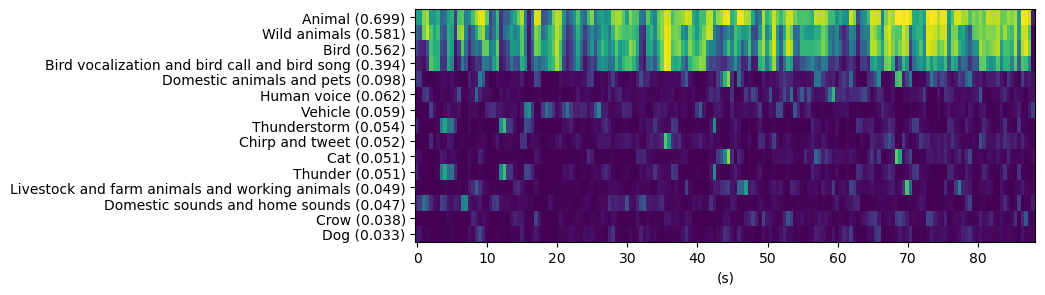# FSD-SINet models released

We are delighted to introduce the FSD-SINet models for sound event classification in Essentia. FSD-SINet is a family of CNN architectures proposed in “Improving Sound Event Classification by Increasing Shift Invariance in Convolutional Neural Networks”  and trained in the FSD50K dataset with techniques to enhance shift invariance. These techniques are:

• Trainable Low-Pass Filters (TLPF) 
• Adaptive Polyphase Sampling (APS) 

The complete list of models supported in Essentia along with their vocabulary is available on Essentia’s site, and the experimental results and implementation details are available in the paper  and the official repository. According to the authors, the fsd-sinet-vgg42-tlpf_aps-1 model featuring TLPF and APS obtained the best evaluation metrics. Additionally, fsd-sinet-vgg41-tlpf-1 is a lighter architecture using TLPF intended for reduced computational cost.

As an example, let’s analyze a field recording and observe the model’s predictions.

The following code performs inference with the fsd-sinet-vgg42-tlpf_aps-1 model:

``````from essentia.standard import MonoLoader, TensorflowPredictFSDSINet

filename = "636921__girlwithsoundrecorder__sounds-from-the-forest.wav"
graph_filename = "fsd-sinet-vgg42-tlpf_aps-1.pb"

model = TensorflowPredictFSDSINet(graphFilename=graph_filename)

activations = model(audio)
``````

Note: Remember to update Essentia before running the code.

These models make predictions each 0.5 seconds by default. Internally, they operate on 10 ms frames, and the number of frames to jump (50 by default) can be controlled with the `patchHopSize` parameter. Additionally, the models can be configured to return embeddings instead of activations by setting the parameter `output="model/global_max_pooling1d/Max"`.

The following code visualizes the top activations using `matplotlib` and the model’s metadata:

``````import json
import matplotlib.pyplot as plt
import numpy as np

def top_from_average(data, top_n=10):
av = np.mean(data, axis=0)
sorting = np.argsort(av)[::-1]
return sorting[:top_n], [av[i] for i in sorting]

# Compute the top-n labels and predictions
top_n, averages = top_from_average(predictions, top_n=15)
top_labels = [labels[i] for i in top_n]
top_labels_with_av = [
f"{label} ({av:.3f})" for label, av in zip(top_labels, averages)
]

top_predictions = np.array([predictions[i, :] for i in top_n])

# Generate plots and improve formatting
matfig = plt.figure(figsize=(8, 3))
plt.matshow(top_predictions, fignum=matfig.number, aspect="auto")

plt.yticks(np.arange(len(top_labels_with_av)), top_labels_with_av)
locs, _ = plt.xticks()
ticks = np.array(locs // 2).astype("int")
plt.xticks(locs[1: -1], ticks[1: -1])
plt.tick_params(
bottom=True, top=False, labelbottom=True, labeltop=False
)
plt.xlabel("(s)")

plt.savefig("activations.png", bbox_inches='tight')
``````As can be seen, the model detects the intermittent bird sounds and some of the backgrounds sounds are identified as Vehicle or Thunder with lower probabilities.

 E. Fonseca, A. Ferraro, X. Serra, “Improving Sound Event Classification by Increasing Shift Invariance in Convolutional Neural Networks,” arXiv:2107.00623, 2021.

 R. Zhang, “Making convolutional networks shift-invariant again,” in International Conference on Machine Learning. PMLR, 2019, pp. 7324–7334.

 A. Chaman and I. Dokmanic, “Truly shift-invariant convolutional neural networks,” in Proceedings of the IEEE/CVF Conference on Computer Vision and Pattern Recognition, 2021, pp. 3773–3783.

 Audio by Freesound user GirlWithSoundRecorder.

[ news ] [ tensorflow ]